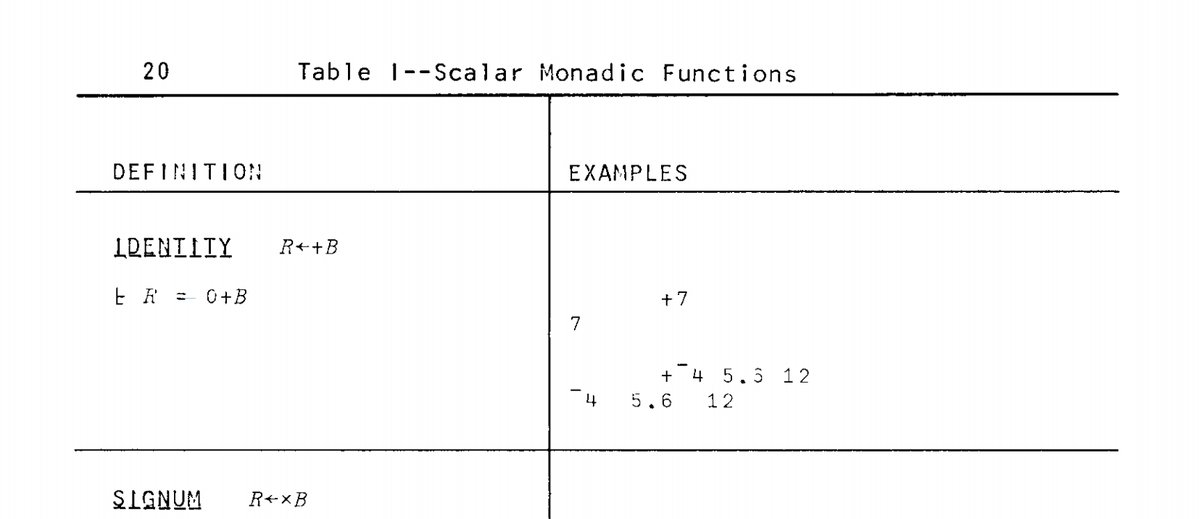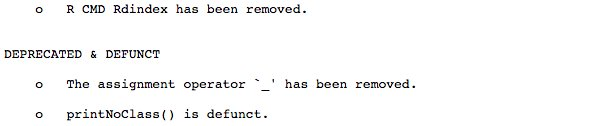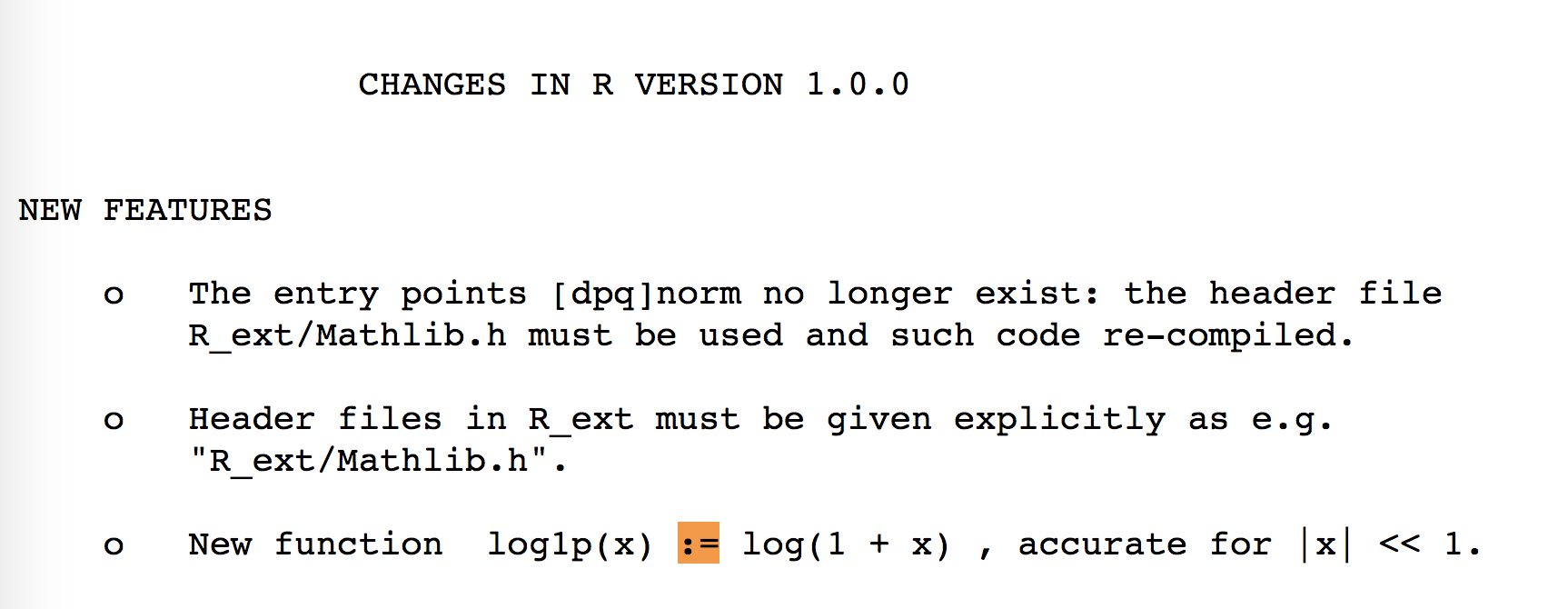# Why do we use arrow as an assignment operator?

In June, I published a little thread on Twitter about the history of the `<-` assignment operator in R. Here is a blog post version of this thread.

## Historical reasons

As you all know, R comes from S. But you might not know a lot about S (I don’t). This language used `<-` as an assignment operator. It’s partly because it was inspired by a language called APL, which also had this sign for assignment.

But why again? APL was designed on a specific keyboard, which had a key for `<-`:At that time, it was also chosen because there was no `==` for testing equality: equality was tested with `=`, so assigning a variable needed to be done with another symbol.Until 2001, in R, `=` could only be used for assigning function arguments, like ```fun(foo = "bar")``` (remember that R was born in 1993). So before 2001, the `<-` was the standard (and only way) to assign value into a variable.

Before that, `_` was also a valid assignment operator. It was removed in R 1.8:(So no, at that time, no snake_case_naming_convention)

Colin Gillespie published some of his code from early 2000, where assignment was made like this :)

The main reason “equal assignment” was introduced is because other languages uses `=` as an assignment method, and because it increased compatibility with S-Plus.

## And today?

Nowadays, there are seldom any cases when you can’t use one in place of the other. It’s safe to use `=` almost everywhere. Yet, `<-` is preferred and advised in R Coding style guides:

One reason, if not historical, to prefer the `<-` is that it clearly states in which side you are making the assignment (you can assign from left to right or from right to left in R):

``````a <-  12
13 -> b
a
``````
``````##  12
``````
``````b
``````
``````##  13
``````
``````a -> b
a <- b
``````

The RHS assignment can for example be used for assigning the result of a pipe

``````library(dplyr)
iris %>%
filter(Species == "setosa") %>%
select(-Species) %>%
summarise_all(mean) -> res
res
``````
``````##   Sepal.Length Sepal.Width Petal.Length Petal.Width
## 1        5.006       3.428        1.462       0.246
``````

Also, it’s easier to distinguish equality comparison and assignment in the last line of code here:

``````c <- 12
d <- 13
e = c == d
f <- c == d
``````

Note that `<<-` and `->>` also exist:

``````create_plop_pouet <- function(a, b){
plop <<- a
b ->> pouet
}
create_plop_pouet(4, 5)
plop
``````
``````##  4
``````
``````pouet
``````
``````##  5
``````

And that Ross Ihaka uses `=` : https://www.stat.auckland.ac.nz/~ihaka/downloads/JSM-2010.pdf

### Environments

There are some environment and precedence differences. For example, assignment with `=` is only done on a functional level, whereas `<-` does it on the top level when called inside as a function argument.

``````median(x = 1:10)
``````
``````##  5.5
``````
``````x
``````
``````## Error in eval(expr, envir, enclos): object 'x' not found
``````
``````median(x <- 1:10)
``````
``````##  5.5
``````
``````x
``````
``````##    1  2  3  4  5  6  7  8  9 10
``````

In the first code, you’re passing `x` as the parameter of the `median` function, whereas the second one is creating a variable x in the environment, and uses it as the first argument of `median`. Note that it works because `x` is the name of the parameter of the function, and won’t work with `y`:

``````median(y = 12)
``````
``````## Error in is.factor(x): argument "x" is missing, with no default
``````
``````median(y <- 12)
``````
``````##  12
``````

There is also a difference in parsing when it comes to both these operators (but I guess this never happens in the real world), one failing and not the other:

``````x <- y = 15
``````
``````## Error in x <- y = 15: could not find function "<-<-"
``````
``````x = y <- 15
c(x, y)
``````
``````##  15 15
``````

It is also good practice because it clearly indicates the difference between function arguments and assignation:

``````x <- shapiro.test(x = iris\$Sepal.Length)
x
``````
``````##
##  Shapiro-Wilk normality test
##
## data:  iris\$Sepal.Length
## W = 0.97609, p-value = 0.01018
``````

And this weird behavior:

``````rm(list = ls())
data.frame(
a = rnorm(10),
b <- rnorm(10)
)
``````
``````##             a b....rnorm.10.
## 1   0.6457433     -0.5001296
## 2   0.2073077     -0.4575013
## 3  -0.4758076     -0.2820372
## 4   0.2568369     -0.4271579
## 5   0.4775034     -1.8024830
## 6   0.9281543     -0.2811589
## 7   0.3622706     -1.5172742
## 8   0.5093346     -1.9805609
## 9  -1.7333491      0.5559907
## 10 -2.0203632      1.9717890
``````
``````a
``````
``````## Error in eval(expr, envir, enclos): object 'a' not found
``````
``````b
``````
``````##   -0.5001296 -0.4575013 -0.2820372 -0.4271579 -1.8024830 -0.2811589
##   -1.5172742 -1.9805609  0.5559907  1.9717890
``````

## Little bit unrelated but

I love this one:

``````g <- 12 -> h
g
``````
``````##  12
``````
``````h
``````
``````##  12
``````

Which of course is not doable with `=`.

## Other operators

Some users pointed out on Twitter that this could make the code a little bit harder to read if you come from another language. `<-` is use “only” use in F#, OCaml, R and S (as far as Wikipedia can tell). Even if `<-` is rare in programming, I guess its meaning is quite easy to grasp, though.

Note that the second most used assignment operator is `:=` (`=` being the most common). It’s used in `{data.table}` and `{rlang}` notably. The `:=` operator is not defined in the current R language, but has not been removed, and is still understood by the R parser. You can’t use it on the top level:

``````a := 12
``````
``````## Error in `:=`(a, 12): could not find function ":="
``````

But as it is still understood by the parser, you can use `:=` as an infix without any %%, for assignment, or for anything else:

```````:=` <- function(x, y){
x\$y <- NULL
x
}
``````
``````##   Sepal.Length Sepal.Width Petal.Length Petal.Width Species
## 1          5.1         3.5          1.4         0.2  setosa
## 2          4.9         3.0          1.4         0.2  setosa
## 3          4.7         3.2          1.3         0.2  setosa
## 4          4.6         3.1          1.5         0.2  setosa
## 5          5.0         3.6          1.4         0.2  setosa
## 6          5.4         3.9          1.7         0.4  setosa
``````

You can see that `:=` was used as an assignment operator https://developer.r-project.org/equalAssign.html :

All the previously allowed assignment operators (<-, :=, _, and <<-) remain fully in effect

Or in R NEWS 1: